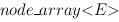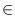Next: Two-Dimensional Node Maps ( Up: Graphs and Related Data Previous: Face Maps ( face_map   Contents   Index

# Two Dimensional Node Arrays ( node_matrix )

Definition

An instance M of the parameterized data type node_matrix<E> is a partial mapping from the set of node pairs V x V of a graph to the set of variables of data type E, called the element type of M. The domain I of M is called the index set of M. M is said to be valid for all node pairs in I. A node matrix can also be viewed as a node array with element type().

#include < LEDA/graph/node_matrix.h >

Creation

 node_matrix M creates an instance M of type node_matrix and initializes the index set of M to the empty set. node_matrix M(const graph_t& G) creates an instance M of type node_matrix and initializes the index set to be the set of all node pairs of graph G, i.e., M is made valid for all pairs in V x V where V is the set of nodes currently contained in G. node_matrix M(const graph_t& G, E x) creates an instance M of type node_matrix and initializes the index set of M to be the set of all node pairs of graph G, i.e., M is made valid for all pairs in V x V where V is the set of nodes currently contained in G. In addition, M(v, w) is initialized with x for all nodes v, wV.

Operations

 void M.init(const graph_t& G) sets the index set of M to V x V, where V is the set of all nodes of G. void M.init(const graph_t& G, E x) sets the index set of M to V x V, where V is the set of all nodes of G and initializes M(v, w) to x for all v, wV. const node_array& M[node v] returns the node_array M(v). const E& M(node v, node w) returns the variable M(v, w). Precondition M must be valid for v and w.

Implementation

Node matrices for a graph G are implemented by vectors of node arrays and an internal numbering of the nodes of G. The access operation takes constant time, the init operation takes time O(n2), where n is the number of nodes currently contained in G. The space requirement is O(n2). Note that a node matrix is only valid for the nodes contained in G at the moment of the matrix declaration or initialization (init). Access operations for later added nodes are not allowed.Next: Two-Dimensional Node Maps ( Up: Graphs and Related Data Previous: Face Maps ( face_map   Contents   Index